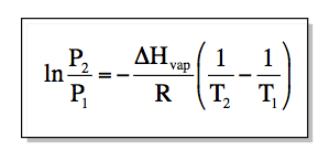# Problem: The vapor pressure of carbon tetrachloride is measured as a function of temperature and the results are tabulated and shown in the table below. Determine the heat of vaporization of carbon tetrachloride from the results.Temperature  (K)Pressure  (torr)25511.326521.027536.828561.529599.0300123.8

###### FREE Expert Solution
• Heat of vaporization can be solved using the Clasius-Clapeyron equation where:• Where P is pressure (any unit will do as long as P1 and P2 have the same unit) and T is temperature in K. ΔHvap is heat of vaporization in J/mol while R is gas constant (8.314 J/mol K)

• Plugging in the values of P and T from the table provided (I'll be using 255 K and 265 K but any will do):

91% (85 ratings)###### Problem Details

The vapor pressure of carbon tetrachloride is measured as a function of temperature and the results are tabulated and shown in the table below. Determine the heat of vaporization of carbon tetrachloride from the results.

 Temperature  (K) Pressure  (torr) 255 11.3 265 21.0 275 36.8 285 61.5 295 99.0 300 123.8